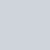# Save NumPy array to file

## 1. Intro

We can learn about creating a NumPy array from plain text files like CSV, TSV in another tutorial. In this tutorial, we will see methods which help us in saving NumPy array on the file system. We can further use them to create a NumPy array.

Few techniques are critical for a data analyst, like saving array in .npy or .npz format. Creation time of NumPy array is very fast from .npy file format, compare to text files like CSV or other. Hence its advisable to save NumPy array in this format, if we wanted to refer them in future.

Python 3.6.5 and NumPy 1.15 is used. Visual Studio Code 1.30.2 used to run iPython interactive codes.

## 2. Save NumPy array as plain text file like CSV

We can save a NumPy array as a plain text file like CSV or TSV. We tend to use this method when we wanted to share some analysis. Most of the analysis passes through multiple steps. Key stakeholders can see the end result with CSV files easily.

We can also provide custom delimiters.

We use numpy.savetxt() method to save a NumPy array as CSV or TSV file.

```#%%
# Saving NumPy array as a csv file
np.savetxt(fname="saved-rain-fall-row-col-names.csv", delimiter=",", X=array_rain_fall)

fname="saved-rain-fall-row-col-names.csv", delimiter=","
)

print("NumPy array: \n", array_rain_fall_csv_saved)
print("Shape: ", array_rain_fall_csv_saved.shape)
print("Data Type: ", array_rain_fall_csv_saved.dtype.name)```

OUTPUT:

```NumPy array:
[[12.  12.  14.  16.  19.  12.  11.  14.  17.  19.  11.  11.5]
[13.  11.  13.5 16.7 15.  11.  12.  11.  19.  18.  13.  12.5]]
Shape:  (2, 12)
Data Type:  float64```

## 3. Save and read NumPy Binary file

We can save the NumPy array as a binary file format using numpy_array.tofile() method. While it is not recommended for cross-machine use for archival and transfer, as it losses the precision and endiness information. It’s better to use .npy or .npz format for the archival and retrieving purpose.

We use numpy.fromfile() method to create a NumPy array from a binary file.

```#%%

# Saving array as binary file and reading it

array_rain_fall.tofile("saved-rain-fall-binary")

array_rain_fall_binary = np.fromfile("saved-rain-fall-binary")

print("NumPy array: \n", array_rain_fall_binary)
print("Shape: ", array_rain_fall_binary.shape)
print("Data Type: ", array_rain_fall_binary.dtype.name)```

OUTPUT:

```NumPy array:
[12.  12.  14.  16.  19.  12.  11.  14.  17.  19.  11.  11.5 13.  11.
13.5 16.7 15.  11.  12.  11.  19.  18.  13.  12.5]
Shape:  (24,)
Data Type:  float64```

## 4. Save and read npy file

We recommend developers to use .npy and .npz files to save NumPy array on disk for easy persistence and fast retrieval. Creating an array using .npy file is faster in comparison to CSV or plain text files.

We use numpy.save() method to save file in .npy format.

numpy.save(file, arr, allow_pickle=True, fix_imports=True)

We create NumPy array from .npy file using numpy.load() method.

```#%%

# Saving array as .npy and reading it

np.save("saved-rain-fall-binary.npy", array_rain_fall)

print("NumPy array: \n", array_rain_fall_npy)
print("Shape: ", array_rain_fall_npy.shape)
print("Data Type: ", array_rain_fall_npy.dtype.name)```

OUTPUT:

```NumPy array:
[[12.  12.  14.  16.  19.  12.  11.  14.  17.  19.  11.  11.5]
[13.  11.  13.5 16.7 15.  11.  12.  11.  19.  18.  13.  12.5]]
Shape:  (2, 12)
Data Type:  float64```

## 5. Save multiple arrays in one npz file

NumPy provides numpy.savez() to save multiple arrays in one file. We can load the .npz file with numpy.load() method.

numpy.savez(file, *args, **kwds)

Combining several NumPy arrays into npz file, results in a faster load of NumPy arrays, comparing it with individual npy files.

```#%%

np.savez("saved-rain-fall-binary.npz", array_rain_fall, np.array([1, 2, 3, 4, 5]))

print("NumPy array 1: \n", array_rain_fall_npz["arr_0"])
print("Shape of Array 1: ", array_rain_fall_npz["arr_0"].shape)
print("Data Type of Array 1: ", array_rain_fall_npz["arr_0"].dtype.name)

print("NumPy array 2: \n", array_rain_fall_npz["arr_1"])
print("Shape of Array 2: ", array_rain_fall_npz["arr_1"].shape)
print("Data Type of Array 2: ", array_rain_fall_npz["arr_1"].dtype.name)```

OUTPUT:

```NumPy array 1:
[[12.  12.  14.  16.  19.  12.  11.  14.  17.  19.  11.  11.5]
[13.  11.  13.5 16.7 15.  11.  12.  11.  19.  18.  13.  12.5]]
Shape of Array 1:  (2, 12)
Data Type of Array 1:  float64
NumPy array 2:
[1 2 3 4 5]
Shape of Array 2:  (5,)
Data Type of Array 2:  int64```

We use numpy.savez_compressed() method to save compressed npz file.

## 6. Conclusion

This tutorial provides useful methods, which you use to optimize your NumPy code further. Save multiple arrays on disk and load them quickly to increase code efficiency and performance.

Please download source code related to this tutorial here. You can run the Jupyter notebook for this tutorial here.

### 1 thought on “Save NumPy array to file”

1.